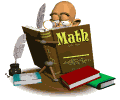• Math

Here are links to websites that you can use to enrich your child's math skills.Math-Basic Facts and Computation
ABCya!Math and other activities. Click on the grade level.
A Plus MathDeveloped to help students improve their math skills interactively. Test your math skills with our Flashcards! Try out the Math Word Find puzzle. See the Worksheets section, where you can print worksheets to practice offline.
Addition Machine Choose a skill level and off you go!
Basket Math Interactive This online, interactive learning is fun, with choices of activities in addition, subtraction, multiplication, division, rounding, order of operations, perimeter, distance, word problems, and more.
Cool Math Games Play some "cool math games" at this site including the classic simulation game Lemonade Stand.
Count Us In Fifteen math games for young students including subtraction bowling and counting sheep.
Division Machine Pick a level! This should not be too hard if you know yourtimes tables.
Dude's Dilemma Fly a helicopter and rescue Dude the dog using math facts. You can choose the operation and the level of difficulty.
Houghton Mifflin Mathematicshas activities for each grade level that offer test prep practice, brain teasers and more.There are also parent and teacher resources.
IKnowthat.com Language arts, math, science, social studies. Check with your librarian because you need the password!
Interactive Math Games Find a variety of different games including basic operations, fractions, telling time, and even directions to make your own.
Mrs. Dell's Multiplication PlaceLots of ways to practice your facts. Try thetimes table test.
Math Cats Covers everything from counting change to the artistic beauty in math. Math Cats' numerous activities include the Polygon Playground, how to make polyhedral ornaments, and math games and math art.
Math Fact Cafe Use flashcard as well as other tools to practice your math facts.
Math LabDesigned for students in grades 3-12 toEXPERIENCE math. Findlinks to math lessons, games, printouts,and other math resources.
Math Magician Various timed tests on the four basic operations. There are many options.
Math Playground is a math learning site for students in grades K-6. It features a large collection of word problems, math games, and logic puzzles.
Multiplication Machine Quick simple practice for your times tables.
Multiplication.com Has games and activities to help you master those multiplication facts.
Multiflyer Students progress through the solar system by correctly answering their multiplication facts from 1 to 12. A multiplication grid is also available as a cool tool.
Primary Games- Math Math games such as Mini-Golf, Carnival Jackpot, Time Clock and Topsy Turvey.
Subtraction Machine Choose a skill level and off you go.